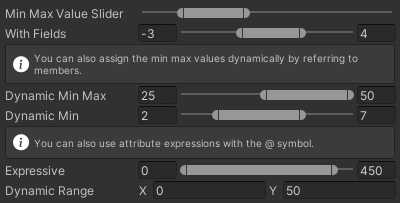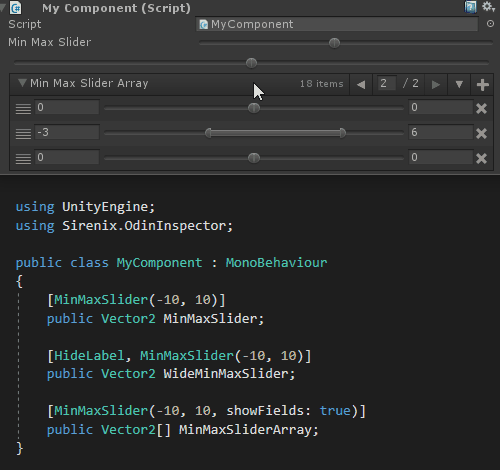• Type Specifics
• Essentials
• Groups
• Buttons
• Misc
• Collections
• Conditionals
• Numbers
• Unity
• Debug
• Meta

# Min Max Slider Attribute

Draw a special slider the user can use to specify a range between a min and a max value. Uses a Vector2 where x is min and y is max.``````[MinMaxSlider(-10, 10)]
public Vector2 MinMaxValueSlider = new Vector2(-7, -2);

[MinMaxSlider(-10, 10, true)]
public Vector2 WithFields = new Vector2(-3, 4);

[InfoBox("You can also assign the min max values dynamically by referring to members.")]
[MinMaxSlider("DynamicRange", true)]
public Vector2 DynamicMinMax = new Vector2(25, 50);

[MinMaxSlider("Min", 10f, true)]
public Vector2 DynamicMin = new Vector2(2, 7);

[InfoBox("You can also use attribute expressions with the @ symbol.")]
[MinMaxSlider("@DynamicRange.x", "@DynamicRange.y * 10f", true)]
public Vector2 Expressive = new Vector2(0, 450);

public Vector2 DynamicRange = new Vector2(0, 50);

public float Min { get { return this.DynamicRange.x; } }

public float Max { get { return this.DynamicRange.y; } }``````